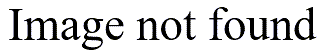# The minimal impact of population size on power and precision

## 2001/01/19

*Dear Professor Mean

• Can you explain why it is okay to have similar sample sizes for populations of very different sizes. For example
• why is it that a sample size for a population of 10 million doesn’t have to be much larger than a sample size for a population of 10 thousand? – Skeptical Sam*

Dear Skeptical,

It is surprising

• but **for the most part
• you disregard the size of the population when you try to estimate an appropriate sample size**. There are some exceptions. Suppose you are trying to sample from the population of all ethical lawyers. The sampling simplifies to deciding which one of the two lawyers you want to chose.

The best analogy I have heard about sampling goes something like: “Every cook knows that it only takes a single sip from a well-stirred soup to determine the taste.” It’s a nice analogy because you can visualize what happens when the soup is poorly stirred.

With regards to why a sample size characterizes a population of 10 million and a population of 10 thousand equally well

• use the soup analogy again. A single sip is sufficient both for a small pot and a large pot.

Finite Population Correction factor (fpc)

When the size of the sample becomes a large fraction of the size of the population

• this analogy no longer holds. In this situation
• we use something called a finite population correction factor (fpc). The finite population correction factor measures how much extra precision we achieve when the sample size become close to the population size.

The formula for fpc is.height="62”}

where N is the size of the population and n is the size of the sample. If fpc is close to 1

• then there is almost no effect. When fpc is much smaller than 1
• then sampling a large fraction of the population is indeed having an effect on precision.

The following table shows what the fpc in four different situations would be.height="125”}

When the sample size is 50

• it does not matter much whether the population is 10 thousand or 10 million. When the sample size, however
• is four thousand
• then we have about 23% more precision with a population of ten thousand than we would for a population of ten million.

A good rule of thumb is to use the fpc when the sample is 10% or more of the population.

Be cautious about using the fpc. Frequently you want to generalize to a larger population than the one you sampled from. You may have restricted the population out of convenience

• but you are interested in more than just the convenient population. This extrapolation will add to the uncertainty of your estimates
• so the last thing you would want to do is to use the fpc to make your confidence intervals narrower.

For example

• you might sample from a set of medical records from February to June of 2002
• but you really are interested in all records
• past
• present
• or future. Or you might sample from patients at your own hospital but you want to generalize to patients at all hospitals. **The finite population correction factor really only applies to “warehouse” type studies
• where you are trying to characterize all the data in a single physical or conceptual location and there is NO interest in data outside of this location.** Warehouse studies are quite common in accounting
• but they are unusual in medical research.

Summary

Uncle Gene wants an explanation of why a sample for a population of 10 million people doesn’t have to be much larger than a sample for a population of 10 thousand people. Professor Mean provides an analogy to taking a single sip from a well stirred soup. He then presents the finite population correction factor and shows that it does not make much of a difference unless your sample is a large fraction of the total population.